UNIT 6 FRACTIONS PERCENTS RATIOS Fractions Percents Ratios

• Slides: 51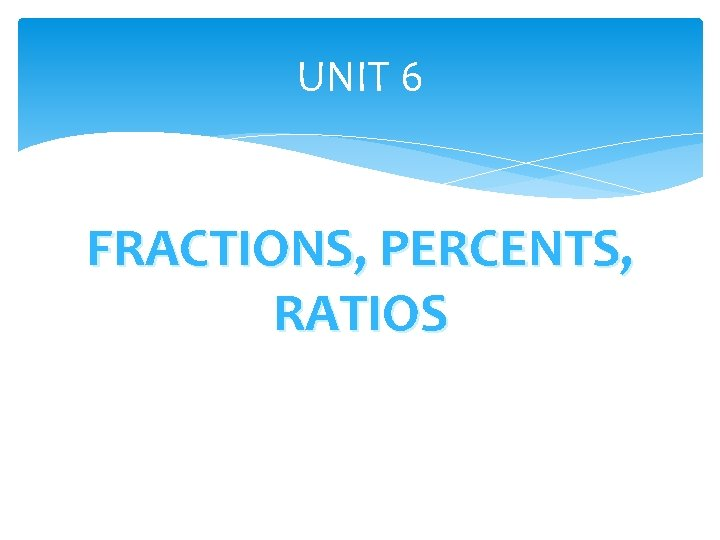UNIT 6 FRACTIONS, PERCENTS, RATIOSFractions, Percents, Ratios Mixed and Improper Fractions (Page 162 -165) Converting Mixed and Improper Fractions (Page 166169) Comparing Mixed and Improper Fractions (Page 171175) Part to Part and Part to Whole Ratios (Page 176 -179) Equivalent Ratios (Page 180 - 183) Percents (Page 186 -189) Comparing Percents, Decimals, and Fractions (Page 190 -193)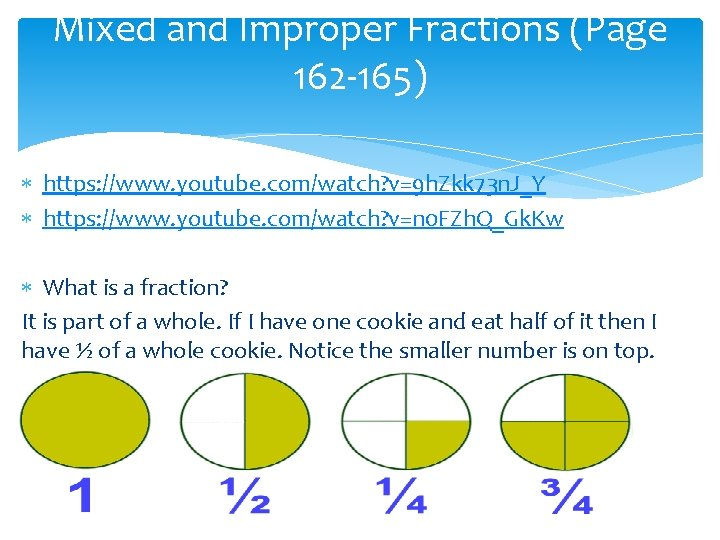Mixed and Improper Fractions (Page 162 -165) https: //www. youtube. com/watch? v=9 h. Zkk 73 n. J_Y https: //www. youtube. com/watch? v=n 0 FZh. Q_Gk. Kw What is a fraction? It is part of a whole. If I have one cookie and eat half of it then I have ½ of a whole cookie. Notice the smaller number is on top.Mixed and Improper Fractions (Page 162 -165)Mixed and Improper Fractions (Page 162 -165)Mixed and Improper Fractions (Page 162 -165) https: //www. youtube. com/watch? v=-55 KA 6 ka. XFY https: //www. youtube. com/watch? v=gg. Yd. Pef 3 Nuk What is a mixed number? It’s when you see a whole number and a fraction together. If I have 2 cookies and I eat half of one then I have 1 and ½ cookies left. 1½ is a mixed number. Count how many wholes you have and then count the fraction.Practice Draw 3¼, 5½, 2⅛, 4⅖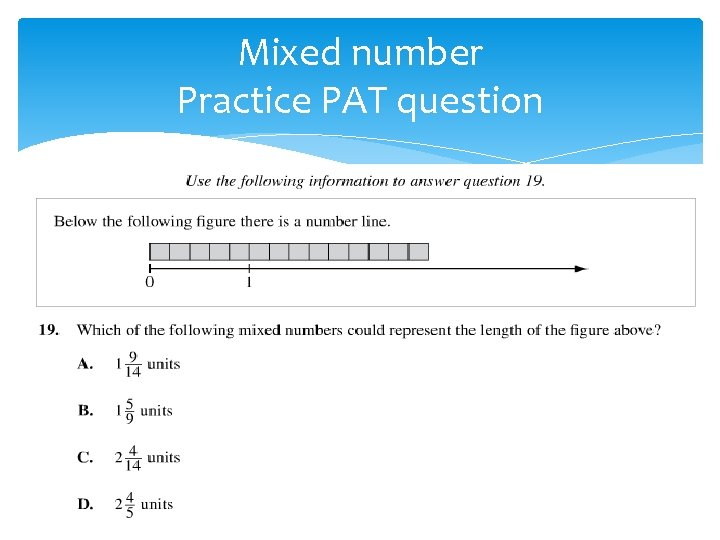Mixed number Practice PAT questionMixed and Improper Fractions (Page 162 -165) https: //www. youtube. com/watch? v=gg. Yd. Pef 3 Nuk What is an improper fraction? A fraction that has a larger numerator than denominator. Which means they are bigger than 1(whole. ) Fractions shouldn’t be larger than a whole that’s what makes them improper.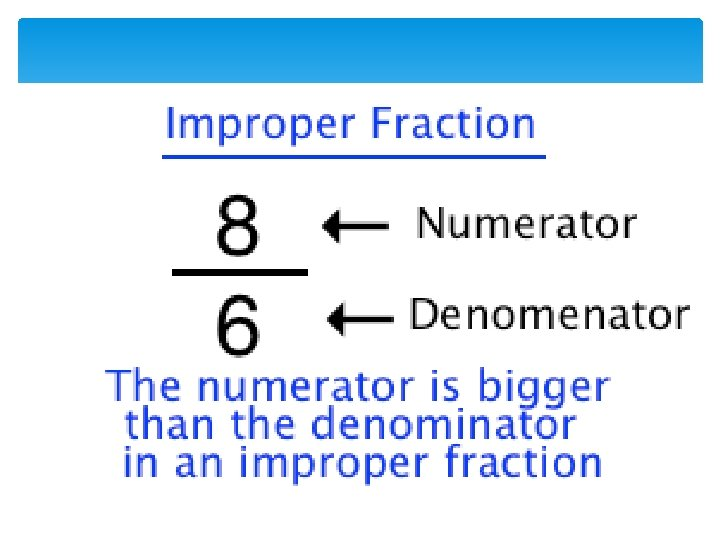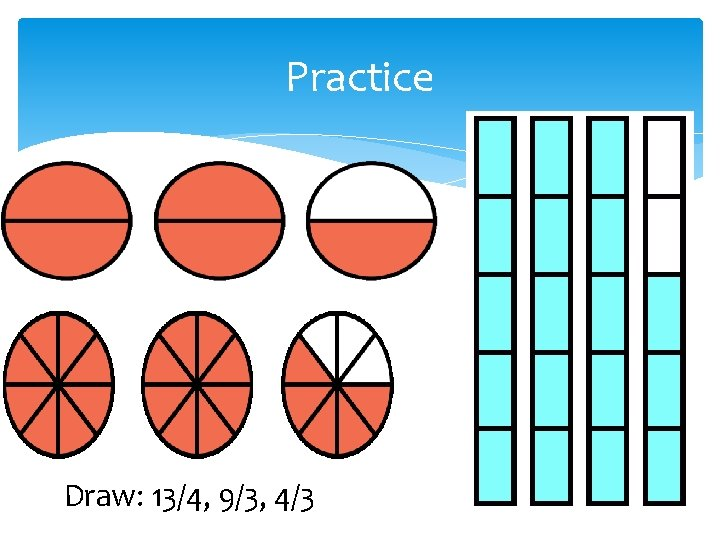Practice Draw: 13/4, 9/3, 4/3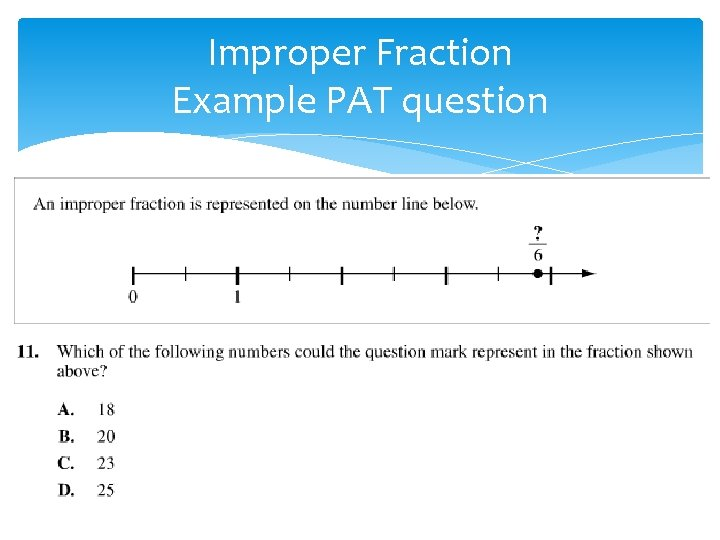Improper Fraction Example PAT question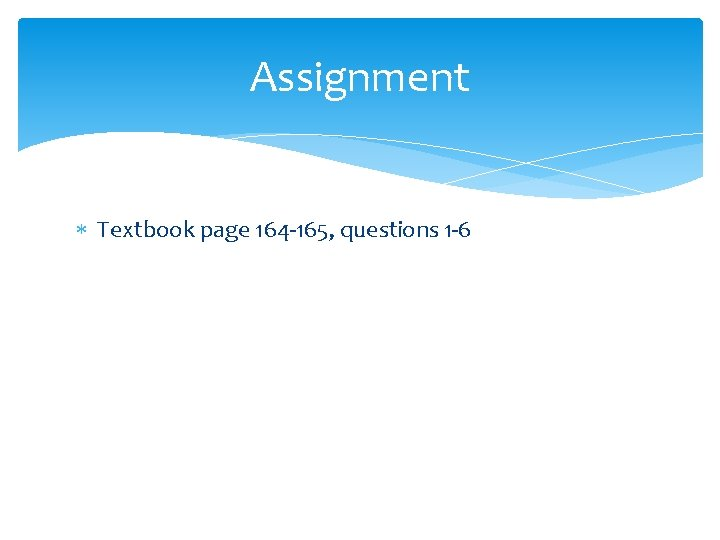Assignment Textbook page 164 -165, questions 1 -6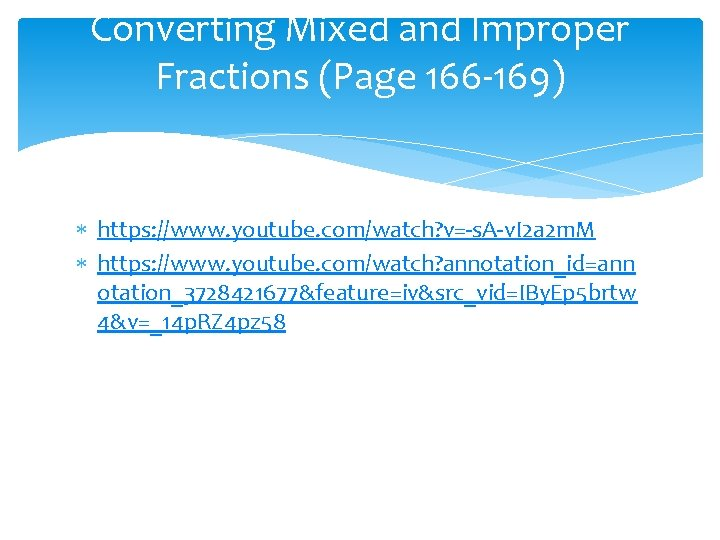Converting Mixed and Improper Fractions (Page 166 -169) https: //www. youtube. com/watch? v=-s. A-v. I 2 a 2 m. M https: //www. youtube. com/watch? annotation_id=ann otation_3728421677&feature=iv&src_vid=IBy. Ep 5 brtw 4&v=_14 p. RZ 4 pz 58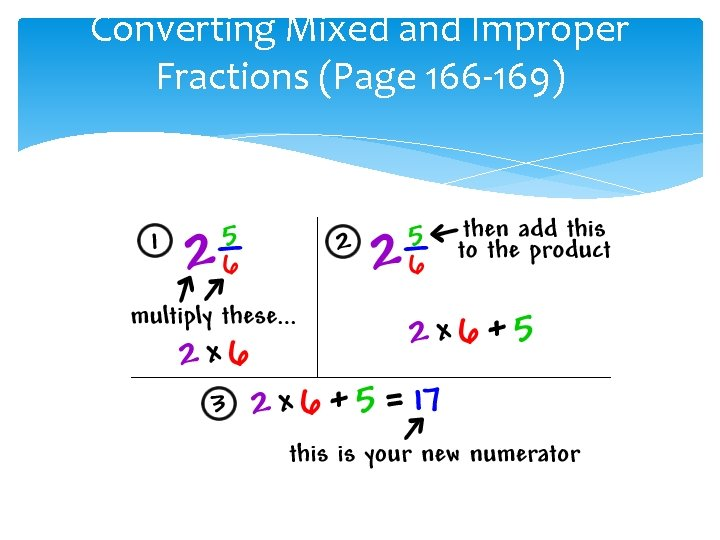Converting Mixed and Improper Fractions (Page 166 -169)Converting Mixed and Improper Fractions (Page 166 -169) To change a mixed number to an improper fraction you multiply the whole number by the denominator and add the numerator. The answer is your new numerator. Remember you always keep your original denominator.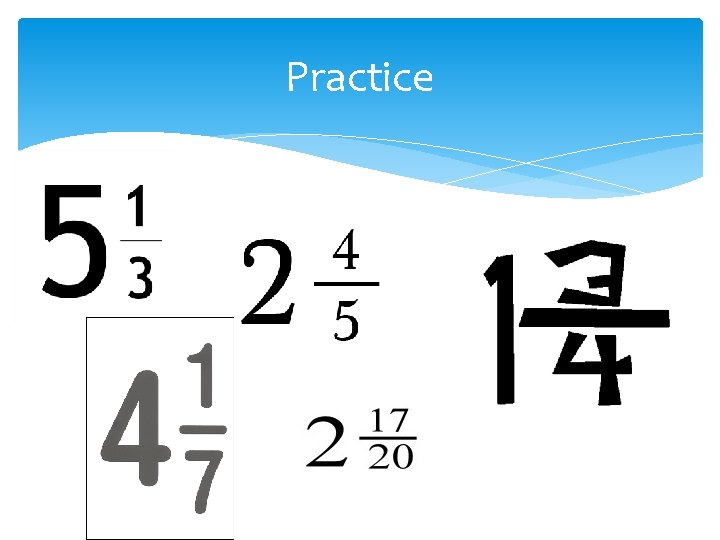PracticeConverting mixed numbers to improper fractions Example PAT questionAssignment Textbook page 168 -169, questions 1 -7 Worksheet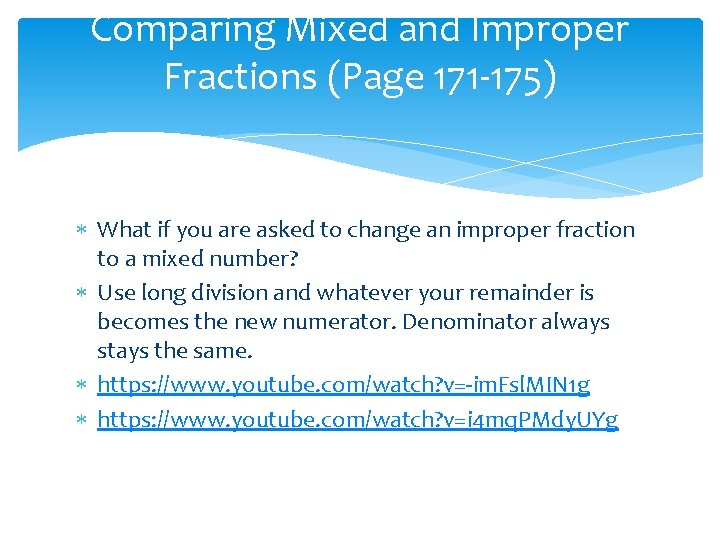Comparing Mixed and Improper Fractions (Page 171 -175) What if you are asked to change an improper fraction to a mixed number? Use long division and whatever your remainder is becomes the new numerator. Denominator always stays the same. https: //www. youtube. com/watch? v=-im. Fsl. MIN 1 g https: //www. youtube. com/watch? v=i 4 mq. PMdy. UYg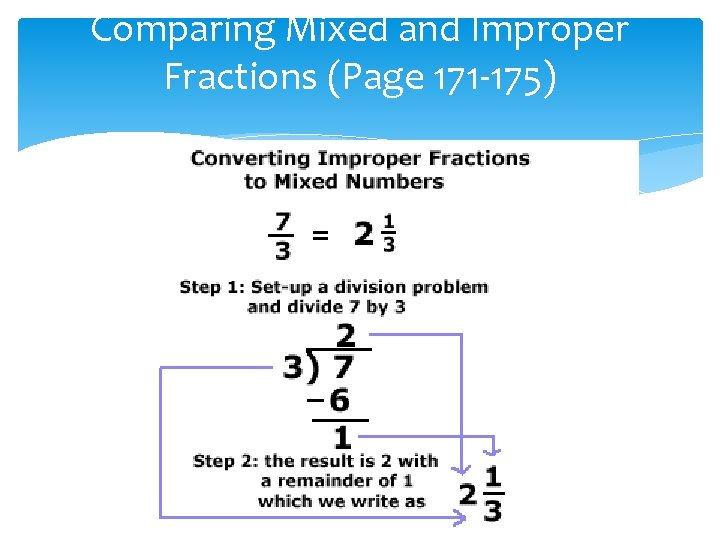Comparing Mixed and Improper Fractions (Page 171 -175)Comparing Mixed and Improper Fractions (Page 171 -175)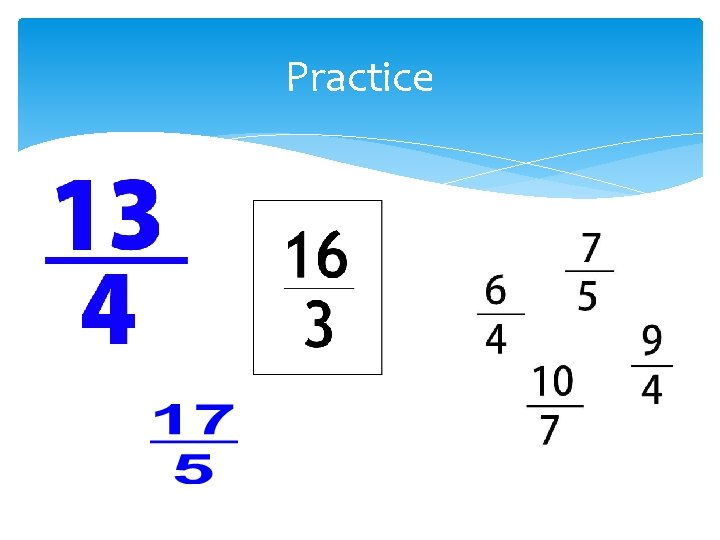Practice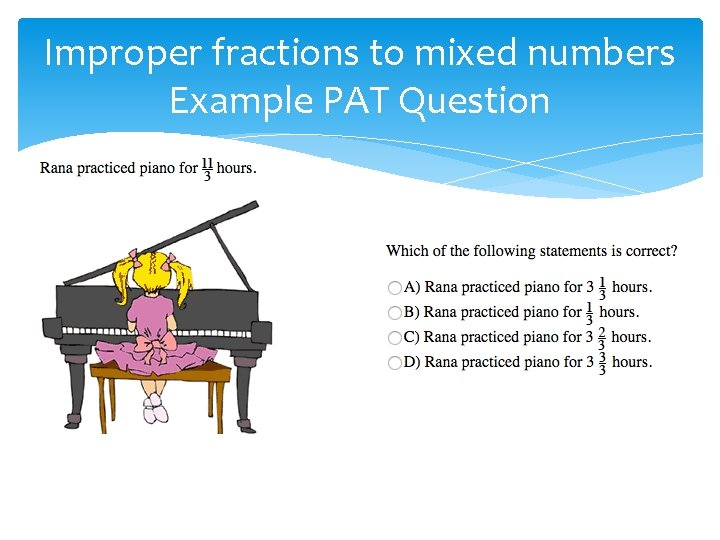Improper fractions to mixed numbers Example PAT QuestionAssignment Worksheet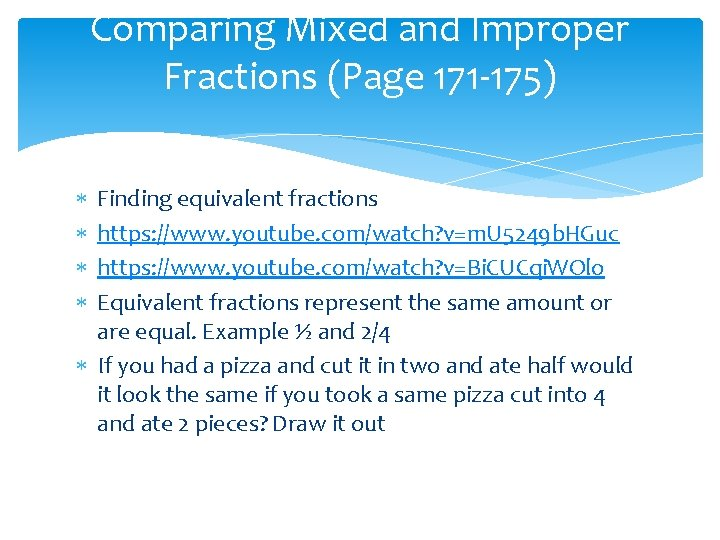Comparing Mixed and Improper Fractions (Page 171 -175) Finding equivalent fractions https: //www. youtube. com/watch? v=m. U 5249 b. HGuc https: //www. youtube. com/watch? v=Bi. CUCqi. WOlo Equivalent fractions represent the same amount or are equal. Example ½ and 2/4 If you had a pizza and cut it in two and ate half would it look the same if you took a same pizza cut into 4 and ate 2 pieces? Draw it outEquivalent Fractions The easiest way to find equivalent fractions to to multiply the denominator and numerator by the same number. Or you can divide the numerator and denominator by the same number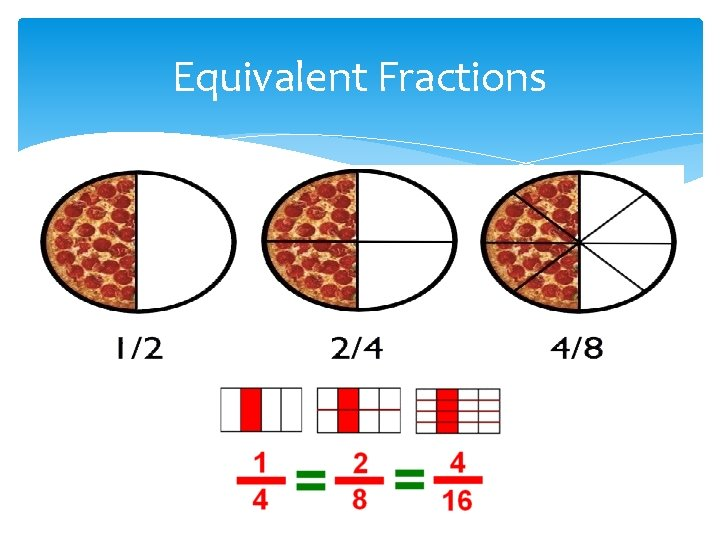Equivalent FractionsPractice Find 3 equivalent fractions to Find equivalent fractions so that the fractions in this pair have the same denominator. and Reduce the following fractions (Hint: Divide) 1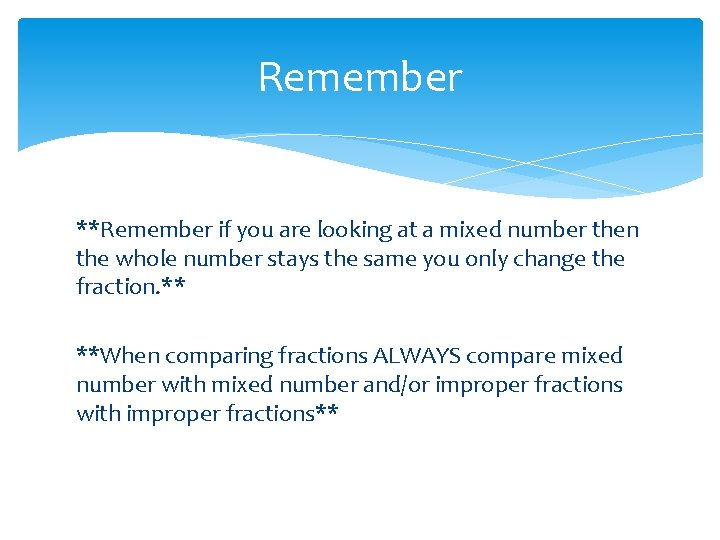Remember **Remember if you are looking at a mixed number then the whole number stays the same you only change the fraction. ** **When comparing fractions ALWAYS compare mixed number with mixed number and/or improper fractions with improper fractions**Comparing Fractions Example PAT questionAssignment Textbook page 173 -175, questions 1 -10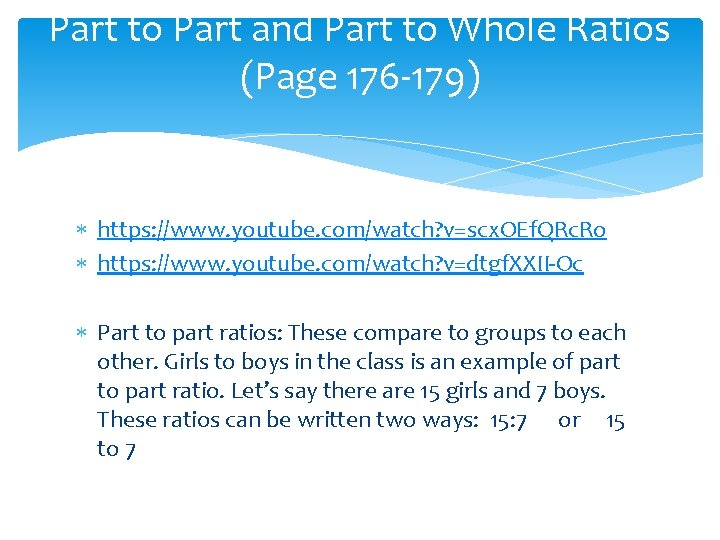Part to Part and Part to Whole Ratios (Page 176 -179) https: //www. youtube. com/watch? v=scx. OEf. QRc. Ro https: //www. youtube. com/watch? v=dtgf. XXII-Oc Part to part ratios: These compare to groups to each other. Girls to boys in the class is an example of part to part ratio. Let’s say there are 15 girls and 7 boys. These ratios can be written two ways: 15: 7 or 15 to 7Part to Part and Part to Whole Ratios (Page 176 -179) Part to whole ratios: These compare part to the whole. For example girls to all student in the class. Let’s say there are 15 girls and 7 boys. Well how many students totally are there? 15 + 7 = 22. These ratios can be written three ways: 15: 22 or 15 to 22 or as a fraction 15/22 **You can only represent a ratio using a fraction if you are comparing part to whole. **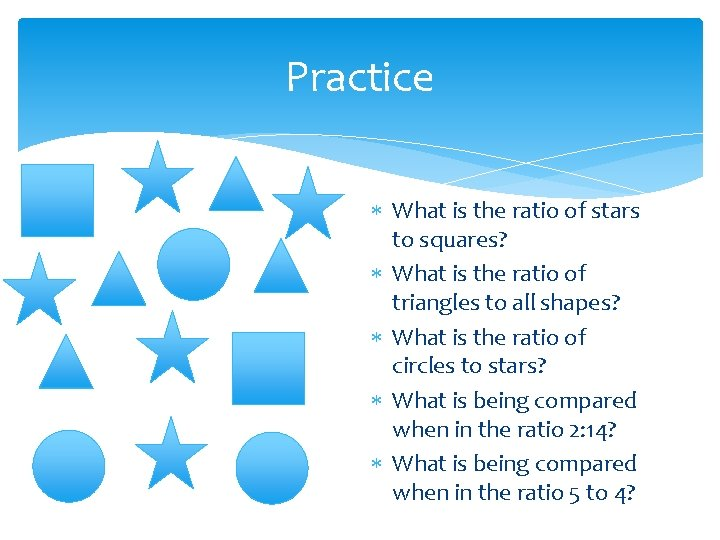Practice What is the ratio of stars to squares? What is the ratio of triangles to all shapes? What is the ratio of circles to stars? What is being compared when in the ratio 2: 14? What is being compared when in the ratio 5 to 4?Ratios Example PAT QuestionsRatios Example PAT QuestionsAssignment Textbook page 178 -179, questions 1 -10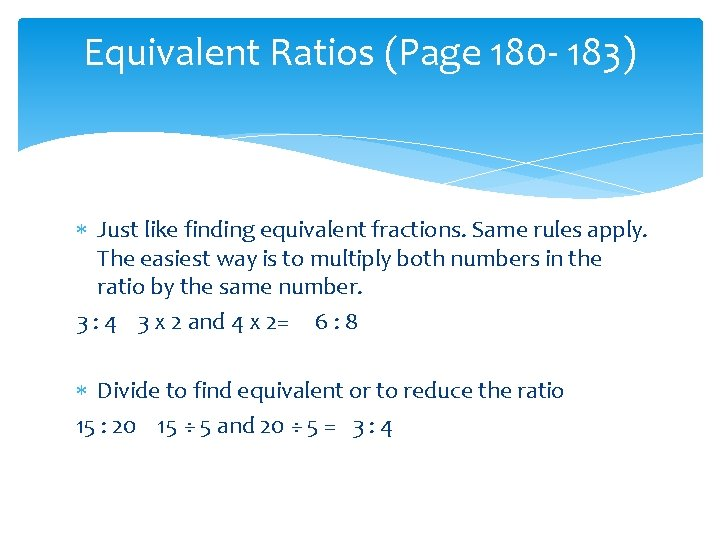Equivalent Ratios (Page 180 - 183) Just like finding equivalent fractions. Same rules apply. The easiest way is to multiply both numbers in the ratio by the same number. 3 : 4 3 x 2 and 4 x 2= 6 : 8 Divide to find equivalent or to reduce the ratio 15 : 20 15 ÷ 5 and 20 ÷ 5 = 3 : 4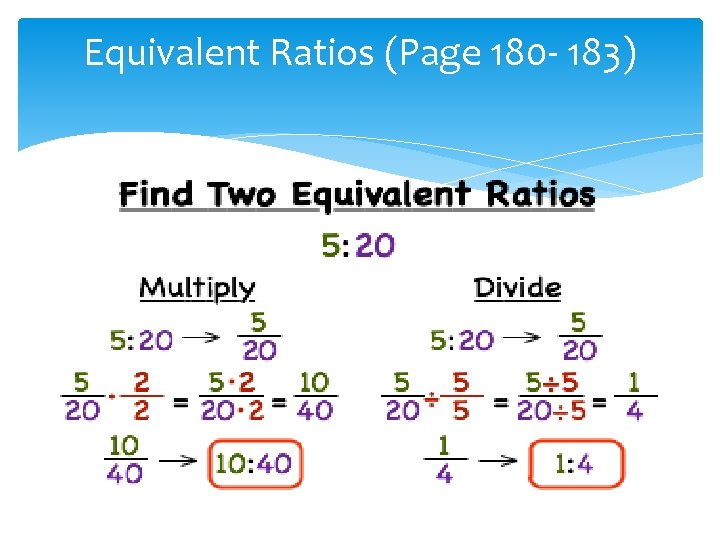Equivalent Ratios (Page 180 - 183)Practice Find 4 equivalent ratios for 5: 6 Find equivalent ratios for the following ratios where one of the terms is 50 3 : 5 10 to 25 7 : 10 1 to 2 ²Reduce the following ratios: 8: 32 4: 16 8: 16 30: 90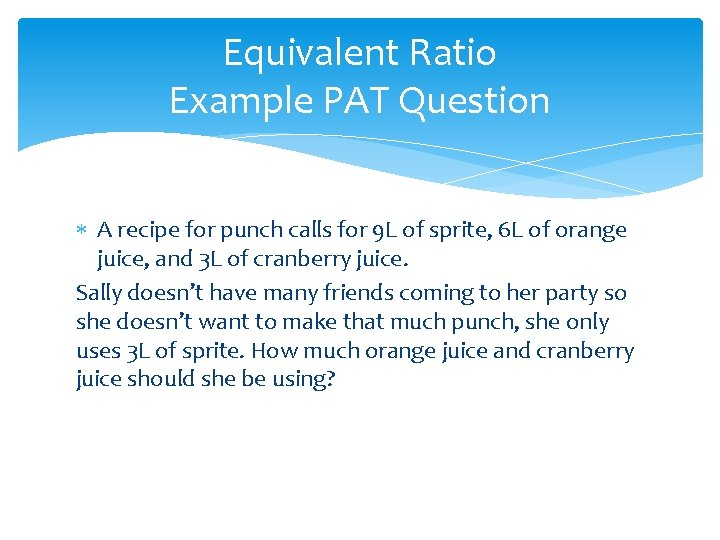Equivalent Ratio Example PAT Question A recipe for punch calls for 9 L of sprite, 6 L of orange juice, and 3 L of cranberry juice. Sally doesn’t have many friends coming to her party so she doesn’t want to make that much punch, she only uses 3 L of sprite. How much orange juice and cranberry juice should she be using?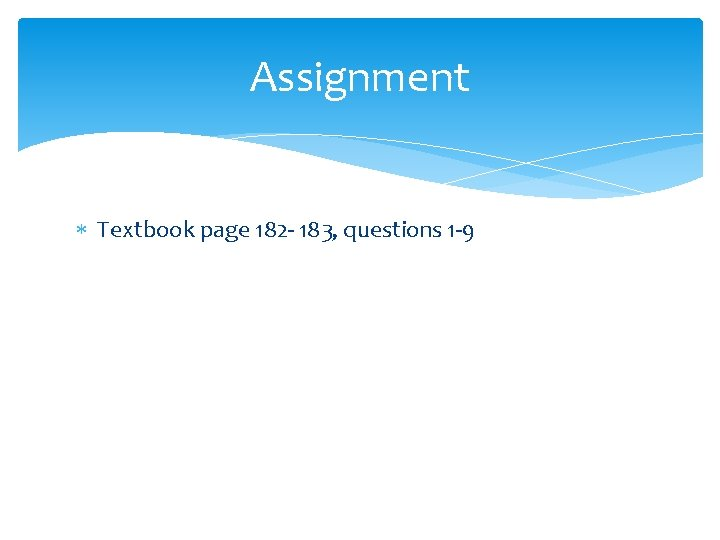Assignment Textbook page 182 - 183, questions 1 -9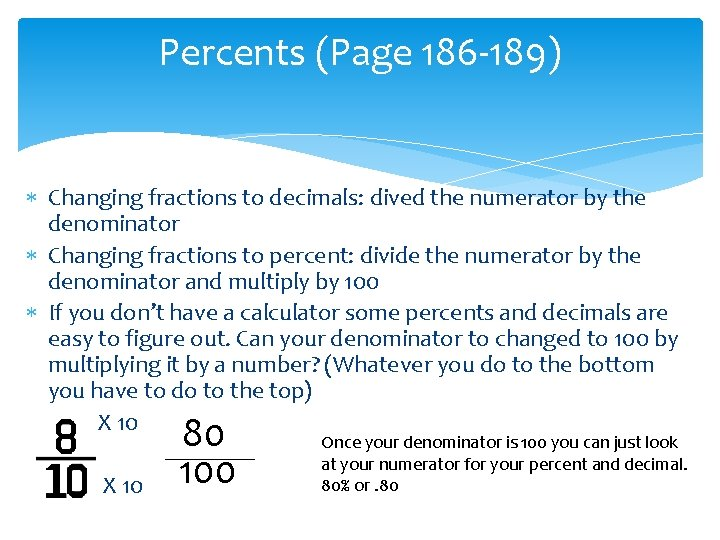Percents (Page 186 -189) Changing fractions to decimals: dived the numerator by the denominator Changing fractions to percent: divide the numerator by the denominator and multiply by 100 If you don’t have a calculator some percents and decimals are easy to figure out. Can your denominator to changed to 100 by multiplying it by a number? (Whatever you do to the bottom you have to do to the top) X 10 80 100 _____ X 10 Once your denominator is 100 you can just look at your numerator for your percent and decimal. 80% or. 80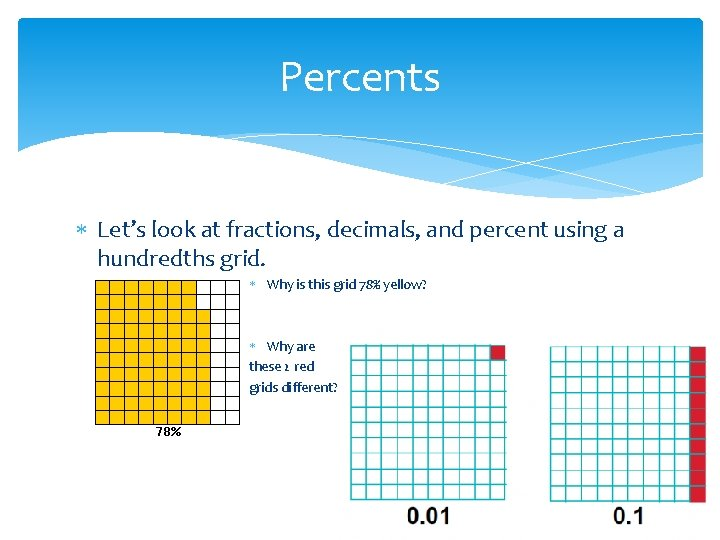Percents Let’s look at fractions, decimals, and percent using a hundredths grid. Why is this grid 78% yellow? Why are these 2 red grids different?Practice What is the percent, fraction, and decimal of the green part of the grid? What is the percent, fraction, and decimal of the white? Write 84% as a decimal and fraction Write 0. 04 as a percent and a fraction Write 30/50 as a percent and decimal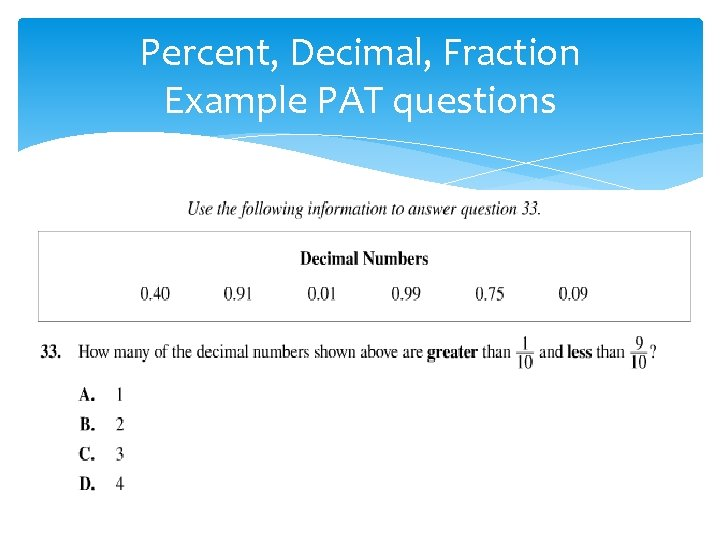Percent, Decimal, Fraction Example PAT questionsPercent, Decimal, Fraction Example PAT questions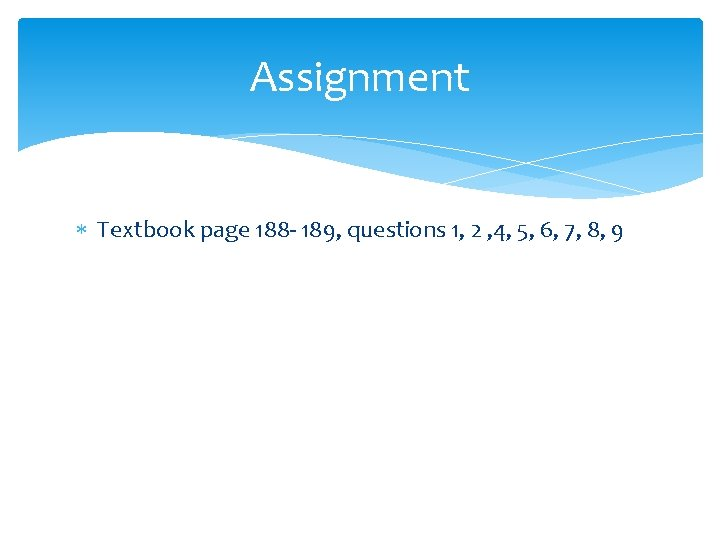Assignment Textbook page 188 - 189, questions 1, 2 , 4, 5, 6, 7, 8, 9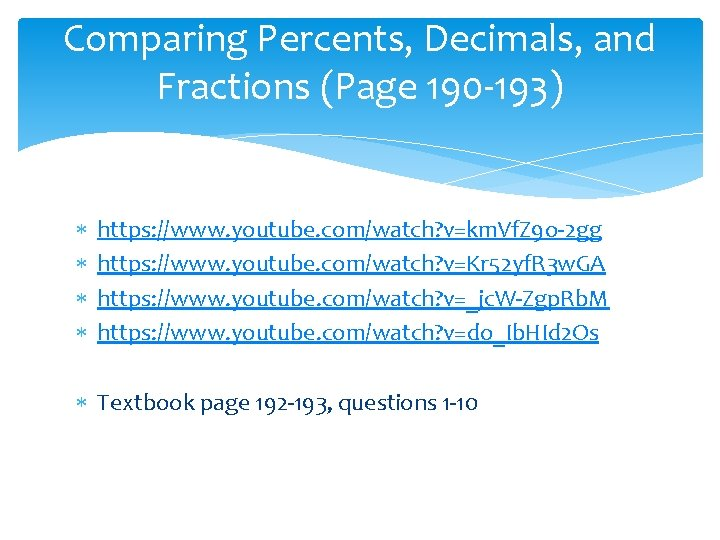Comparing Percents, Decimals, and Fractions (Page 190 -193) https: //www. youtube. com/watch? v=km. Vf. Z 9 o-2 gg https: //www. youtube. com/watch? v=Kr 52 yf. R 3 w. GA https: //www. youtube. com/watch? v=_jc. W-Zgp. Rb. M https: //www. youtube. com/watch? v=do_Ib. HId 2 Os Textbook page 192 -193, questions 1 -10GAMES http: //mathsnacks. com/ratio-rumble. html http: //www. mathplayground. com/ASB_Ratio. Stadium. html http: //www. sheppardsoftware. com/mathgames/fractions/math man_improper_fractions. htm http: //www. mathplayground. com/fractions_mixed. html http: //www. mathplayground. com/fractions_improper. html http: //www. sheppardsoftware. com/mathgames/fractions/memo ry_fractions 3. htm http: //www. sheppardsoftware. com/mathgames/fractions/memo ry_equivalent 1. htm http: //www. mathplayground. com/Decention. html http: //www. topmarks. co. uk/maths-games/7 -11 -years/fractionsand-decimals